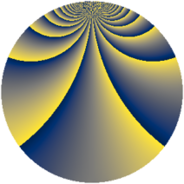# Properties

 Label 2001.2.vLevel $2001$ Weight $2$ Character orbit 2001.v Rep. character $\chi_{2001}(86,\cdot)$ Character field $\Q(\zeta_{22})$ Dimension $2360$ Sturm bound $480$

# Related objects

## Defining parameters

 Level: $$N$$ $$=$$ $$2001 = 3 \cdot 23 \cdot 29$$ Weight: $$k$$ $$=$$ $$2$$ Character orbit: $$[\chi]$$ $$=$$ 2001.v (of order $$22$$ and degree $$10$$) Character conductor: $$\operatorname{cond}(\chi)$$ $$=$$ $$2001$$ Character field: $$\Q(\zeta_{22})$$ Sturm bound: $$480$$

## Dimensions

The following table gives the dimensions of various subspaces of $$M_{2}(2001, [\chi])$$.

Total New Old
Modular forms 2440 2440 0
Cusp forms 2360 2360 0
Eisenstein series 80 80 0

## Trace form

 $$2360q - 268q^{4} - 8q^{6} - 44q^{7} - 10q^{9} + O(q^{10})$$ $$2360q - 268q^{4} - 8q^{6} - 44q^{7} - 10q^{9} - 28q^{13} - 276q^{16} + 140q^{24} - 264q^{25} - 44q^{28} - 22q^{30} - 22q^{33} - 44q^{34} + 36q^{36} - 22q^{42} - 56q^{49} - 22q^{51} + 112q^{52} - 94q^{54} - 88q^{57} + 48q^{58} + 88q^{63} - 224q^{64} - 88q^{67} - 48q^{78} + 78q^{81} + 104q^{82} + 121q^{87} + 132q^{88} - 272q^{93} - 208q^{94} - 56q^{96} + O(q^{100})$$

## Decomposition of $$S_{2}^{\mathrm{new}}(2001, [\chi])$$ into newform subspaces

The newforms in this space have not yet been added to the LMFDB.